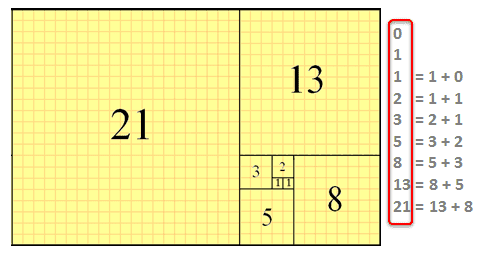JavaForQAFibonacci series numbers – A particular sequence of integers

logic –

First two numbers are 0 and 1, then next subsequent integer will be sum of the previous 2 numbers

let’s see few easy ways to achieve this –

```public static void main1(String[] args) {
int max = 10;
int prev = 0;
int next = 1;
int sum = 1;
System.out.println(prev);
for(int i = 1; i < max; i++)
{
System.out.println(sum);
sum = prev + next;
prev = next;
next = sum;
}
}```

[or]

```public static void main(String[] args) {
int max = 10;
int a = 0;
int b = 1;
for (int i = 0; i < max; i++)
{
System.out.println(a);
a = a+b;
b = a-b;
}
}```

Output:

0
1
1
2
3
5
8
13
21
34